UPSC  >  Test: Line Charts- 1

# Test: Line Charts- 1 - UPSC

Test Description

## 5 Questions MCQ Test CSAT Preparation - Test: Line Charts- 1

Test: Line Charts- 1 for UPSC 2023 is part of CSAT Preparation preparation. The Test: Line Charts- 1 questions and answers have been prepared according to the UPSC exam syllabus.The Test: Line Charts- 1 MCQs are made for UPSC 2023 Exam. Find important definitions, questions, notes, meanings, examples, exercises, MCQs and online tests for Test: Line Charts- 1 below.
Solutions of Test: Line Charts- 1 questions in English are available as part of our CSAT Preparation for UPSC & Test: Line Charts- 1 solutions in Hindi for CSAT Preparation course. Download more important topics, notes, lectures and mock test series for UPSC Exam by signing up for free. Attempt Test: Line Charts- 1 | 5 questions in 10 minutes | Mock test for UPSC preparation | Free important questions MCQ to study CSAT Preparation for UPSC Exam | Download free PDF with solutions
 1 Crore+ students have signed up on EduRev. Have you?
Test: Line Charts- 1 - Question 1

### Instructions: The following chart gives the average of marks in six subjects scored by 25 students in three different exams conducted in an academic year.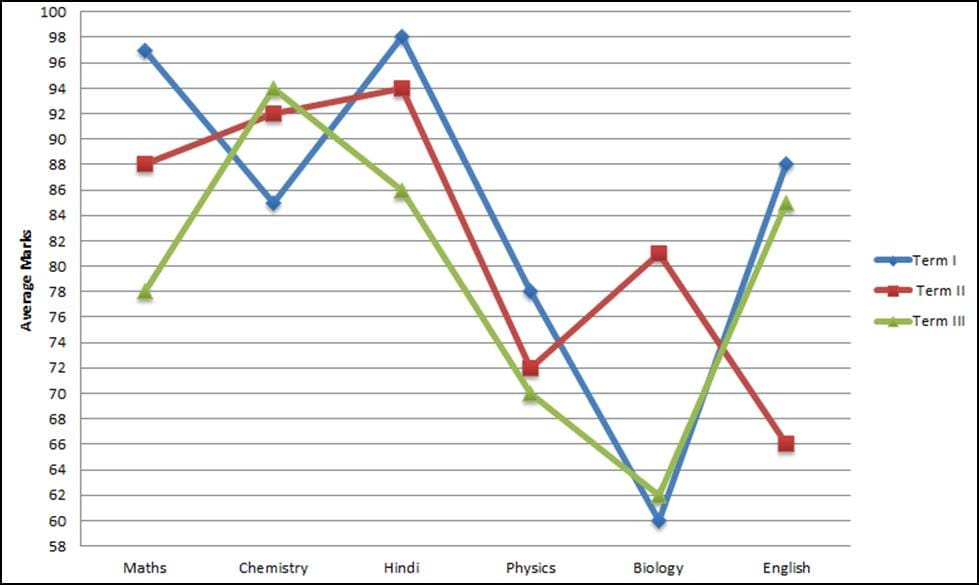The maximum marks of the exam is 100 and a student who scores at least 35 marks is considered as passed. Q. What could be the maximum number of students that failed in all the exams in all the three terms?

Detailed Solution for Test: Line Charts- 1 - Question 1

The number of students who failed in all the six subjects in all the three terms would be limited by the highest average among all sections in all terms.

The highest average is for Hindi in term I which is 98.

The total marks of 25 students for Hindi in term I = 98 x 25 = 2450

If we assume 1 student fails, then maximum marks of the other 24 students = 2450 – 34 = 2416
which is not possible.

Thus, no student can fail in all six subjects in all three terms.

Test: Line Charts- 1 - Question 2

### Instructions: The following chart gives the average of marks in six subjects scored by 25 students in three different exams conducted in an academic year.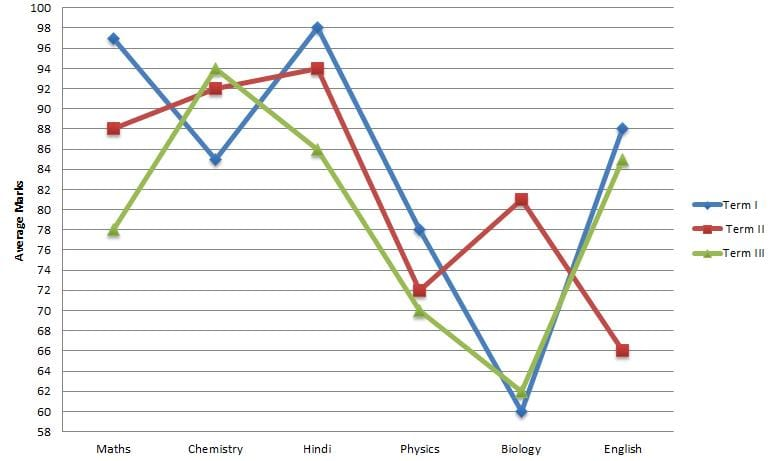The maximum marks of the exam is 100 and a student who scores at least 35 marks is considered as passed. Q. The number of students who failed in all the subjects in the IInd term cannot be more than?

Detailed Solution for Test: Line Charts- 1 - Question 2

The number of students failed will be minimum for the subject with the maximum average marks.
For the II term, Hindi has the maximum average marks.
The total marks scored by all the 25 students in Hindi = 25 x 94 = 2350.
If we assume 3 students failed, then the maximum total marks of the remaining 22 students =2350 – 34×3 = 2248.
But since maximum marks for a subject is only 100, this is not possible.
If we assume 2 students failed, then the maximum total marks of the remaining 23 students = 2350 – 34×2 = 2282 which is possible. Thus, the maximum number of students who have failed in all the subjects in the second term = 2

Test: Line Charts- 1 - Question 3

### Instructions: The following chart gives the average of marks in six subjects scored by 25 students in three different exams conducted in an academic year.The maximum marks of the exam is 100 and a student who scores at least 35 marks is considered as passed. Q. The number of students that scored more than 80 marks in all the subjects in all the term cannot be more than

Detailed Solution for Test: Line Charts- 1 - Question 3

The number of students that scored more than 80 in all subjects in all the terms will be limited by the subject having the lowest average which is 60 for Biology in the first term.
The total score of all the sixty students = 60 x 25 = 1500
Thus, the maximum number of students who scored greater than 80 in all the six subjects for all the terms = 1500/80 = 18.75 i.e 18 students.

Test: Line Charts- 1 - Question 4

Instructions: Refer to the following graphs and answer the given questions:

Graph I gives the percentage of people committing crimes each year in Boselvania and graph II gives the percentage increase in the population of Boselvania each year compared to the previous year.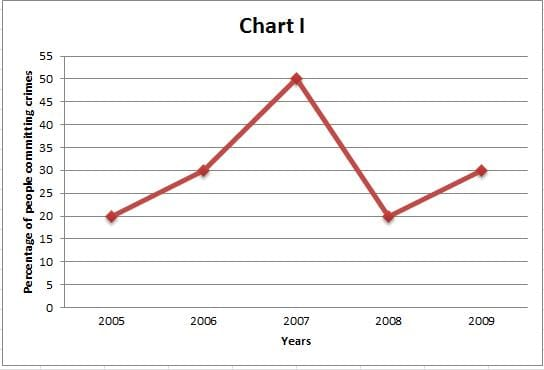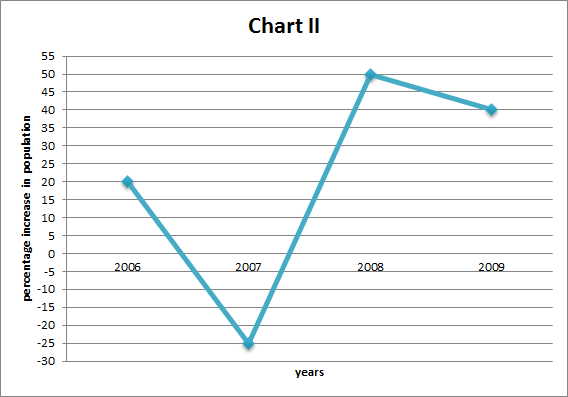Q. In which year among the given years(from 2005-2009) was the number of people that committed crimes the the second lowest?

Detailed Solution for Test: Line Charts- 1 - Question 4

For ease of calculation let’s assume the number of people in Boselvania in 2005 is 100.

In Graph II, the percentage increase in 2006 is 20%.

Thus, the population would be 100×1.2 = 120 in 2006.

Also, the percentage crimes in Boselvania in 2006 is 30%.

Thus, the number of crimes in Boselvania is 120x.3 = 36.

In this way, the table can be formed for all the given years.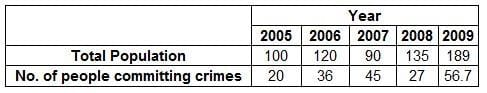From the table, we can see that the number of people committing crimes was second lowest in 2008

Test: Line Charts- 1 - Question 5

Instructions: Refer to the following graphs and answer the given questions:

Graph I gives the percentage of people committing crimes each year in Boselvania and graph II gives the percentage increase in the population of Boselvania each year compared to the previous year.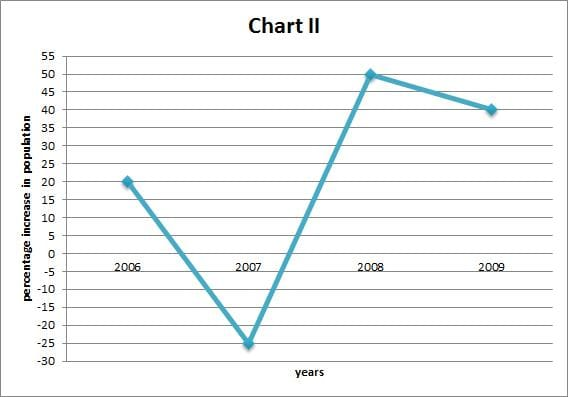Q. In which year among the given years (2005-2009) was the population in Boselvania the third highest?

Detailed Solution for Test: Line Charts- 1 - Question 5

For ease of calculation let’s assume the number of people in Boselvania in 2005 is 100.

In Graph II, the percentage increase in 2006 is 20%.

Thus, the population would be 100×1.2 = 120 in 2006.

Also, the percentage crimes in Boselvania in 2006 is 30%.

Thus, the number of crimes in Boselvania is 120x.3 = 36.

In this way, the table can be formed for all the given years.From the table, we can see that the population was third highest in the year 2006.

## CSAT Preparation

197 videos|151 docs|200 tests
Information about Test: Line Charts- 1 Page
In this test you can find the Exam questions for Test: Line Charts- 1 solved & explained in the simplest way possible. Besides giving Questions and answers for Test: Line Charts- 1, EduRev gives you an ample number of Online tests for practice

## CSAT Preparation

197 videos|151 docs|200 tests

### How to Prepare for UPSC

Read our guide to prepare for UPSC which is created by Toppers & the best Teachers